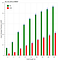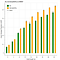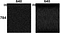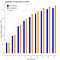# Efficient nearest neighbors inspired by the fruit fly brain

It is quite rare to come across an idea so relevant to machine learning and yet so simple that it can be implemented in a few minutes. So, I was very excited when I came across this paper in Science by Dasgupta et. al. Searching for similar objects in a high dimensional space, such as similar images or documents in a corpus is an important task in data science and machine learning. This paper presented an algorithm inspired by the smell processing circuit of fruit flies, which is 20x faster than commonly used locality sensitive hashing (LSH). In this post, I will describe this algorithm, show the results I got by implementing it and a variant of the approach which I empirically found to work better.

Now, I will give a brief introduction to LSH. If you are already familiar with it, you can jump to the next section. The simplest algorithm for similarity search is k-nearest neighbors but, as you know, it does not scale well both with the dimensions of the inputs as well as the amount of data as the search space becomes large. Locality Sensitive Hashing is a well known algorithm which seeks to reduce the search space by converting a high dimensional vector, V of dimensionality d into a low dimensional binary hash, h of dimensionality m, where d>>m. I will use the MNIST dataset for examples and results in this post. In MNIST each image is 28x28 i.e. d=784. We may convert the images into a m=16 dimensional hash. The hashes are gnerated by projecting the input vectors Vi’s (i.e. taking the dot product) on a small number (m) of random vectors, Uj’s (j=1:m) in the high dimensional space. Generally, these vectors are generated randomly, although other approaches using, for example, PCA can do better. The hash for a vector Vi contains m binary digits and is generated by taking the sign of the dot product of the vector Vi with all Uj’s and setting the j’th bit to a 0 or 1 depending on the sign of the dot product. LSH assigns the same hash to vectors which are ‘close enough’ in the d dimensional space. So, to find the nearest neighbors to a query vector, we need to search only points which have the same hash (or very similar hash, differing by a few bits) as the query vector.

# The New algorithm

The proposed algorithm differs from usual LSH in three ways:

1. The projection vectors are sparse binary vectors (i.e. their directions are aligned with the axes) as opposed to usual LSH which uses random directions. Such sparsity reduces the time complexity of taking the dot products.

2. The dimensionality of hashing space is much higher than the input space i.e. d<<m. This means we have many more vectors in high dimensional space (and therefore many more dot products) as compared to usual LSH but we can afford to do this since each dot product is also much more efficient.

3. The hashing is performed on the basis of which dimensions are most active. Basically, the top 5% of the m dot products are assigned a 1 and all other locations are assigned a 0.

## The Biological analogy

The inspiration for this algorithm comes from the olfactory (smell processing) circuit of fruit flies. The fruit fly circuit has 50 neurons called Oral Receptor Neurons (ORNs) which receive inputs from the external environment and the circuit is tasked with recognizing whether the inputs from the ORNs correspond to a previously known smell. The brain of the fly accomplishes this by first zero-centering the inputs from ORNs into projection neurons (PNs) and then encoding the outputs from PNs into about 2000 Kenyon Cells (KCs) as shown in the illustration below:

In terms of the earlier notation, input vectors of d=50 dimensions are mapped into hashes of m=2000 dimensions. There are three notable aspects of this mechanism. First, the number of neurons in the encoding layer is much larger than the number of input neurons. Second, each KC connects to only about 10% (6 out of 50) of PNs. The weights on these connections are all 1s and other weights are all 0s. This is important because all that a KC needs to do is add up inputs from all the PNs it is connected to and save the cost of multiplication involved in taking the dot product. Third, the most active KCs inhibit other neurons from firing at all. In the above figure, the neurons denoted by solid circles are the most active and they suppress the output of other neurons which are not so active. Overall, only the top 5% (about 100) of all KCs fire and the rest are zero. Thus, the way a fruit fly recognizes the smell is based on which of the 100 KCs fired. The number of neurons firing is called the hash length k in the paper. Since only 5% of the neurons fire, m=20k.

We can think of the usual LSH as a densely connected two layer neural network with d neurons in the input layer and m neurons in the output layer (d>>m) with a step function (f(x)=1 if x>0 else 0) as the activation function. The new Fly inspired LSH can be seen as a sparsely connected network with ‘argsort’ as the activation i.e. f(x)=1 if x∊ top 5% of {xi} else 0.

## Efficiency of generating the hash

It is important to note that to generate a hash of m dimensions, usual LSH performs (d+d)*m computations i.e. d products and d sums for each of the m vectors, whereas the fly algorithm performs only 0.1d*m computations, since each neuron is connected to only 0.1d input neurons. The fly algorithm skips the product step since all the weights are 1 and only 0.1d sums are required. Thus, for the same number of hash dimensions the fly algorithm is 20 times faster (2d*m v/s 0.1d*m). For a fair comparison of performance, I followed the procedure from the paper and used the same computational budget for both algorithms. Thus, I set the hash length k of Fly LSH equal to the hash dimensions m of usual LSH.

# Implementation and Results

Implementing this in Python was pretty easy. I used MNIST dataset and followed the same procedure as used in the paper. All the input vectors were pre-processed to have zero mean. I used a subset of 10,000 points from the dataset and calculated the average precision of LSH and ‘Fly LSH’ for predicting the nearest 200 vectors for each query vector. This was done for 1000 queries and the mean average precision (mAP) was calculated. Here are the results:It works as advertised! I got 3~4x higher mAP scores from Fly LSH for the same computational budget.

It is interesting to note that all the three differences from conventional LSH do not contribute equally to the gains in performance. For example, in all cases above, the dimensionality of hashing space m=20*k. So, d(=784) is not less than m (=40~640). Therefore, dimensionality is not so important for performance but the sparsity of vectors and those of activations are very important for good performance. Here, I used the same computational budget for both algorithms to generate hashes and got better performance from the fly algorithm. In the paper they show that if the number of hashing dimensions for both algorithms are kept same, one gets the same performance but the fly algorithm is 20 times faster.

## Can we do better?

In the previous graph, the m sparse vectors for taking projections were generated randomly. Could we do better if the vectors were somehow learnt from the data and not just random. The paper hints at this but does not show any results. One possible idea is that if the binary projections were taken along directions which are most relevant to encoding the information from d dimensions to m dimensions, then these more relvant dimensions may provide better hashes. I tested this idea by training an autoencoder and choosing the sparse vectors along dimensions with the most significant weights learnt by the encoder. This tends to improve the performance:Learning the directions along which to take projections improves the performance of Fly LSH

It is also interesting to visualize the dimensions which the autoencoding fly LSH learnt to be most important as shown below:For hash length k=32 (a) Directions assigned randomly, and (b) Directions learnt by the autoencoder. Both images are 784x640 matrices. In (b) the highest 10% weights along each column were binarized to 1.

The reason for the bands in figure (b) above is related to the nature of the MNIST dataset since the digits occur usually in the middle of the image. Although there is a computational cost to train the autoencoder, it can be well worth the effort if precision is important for our application.

We could take this idea further and encourage the network to learn to encode the inputs in a sparse way i.e. such that most of the weights are zero and most of the heavy lifting for encoding the inputs is performed by a few weights. This is different from a sparse autoencoder, which constrains the activations of the neurons but allows the weights to take any values. Although this approach feels promising, I have not been able to get it to work better than the dense autoencoder as shown below. But this is still a work in progress. I would highly appreciate any suggestions/pull requests on GitHub for ideas which could improve the performance of this new LSH algorithm.An autoencoder with sparse weights performs slightly worse on MNIST. I appreciate any ideas to improve this.

# TL;DR

There is a new algorithm for searching nearest neighbors in high dimensional spaces. It works very well. You should check it out. Code is available on GitHub. Suggestions for improvement are welcome.

Written by

## Jaiyam Sharma

#### Blogs about replicating research papers in machine learning

Welcome to a place where words matter. On Medium, smart voices and original ideas take center stage - with no ads in sight. Watch
Follow all the topics you care about, and we’ll deliver the best stories for you to your homepage and inbox. Explore
Get unlimited access to the best stories on Medium — and support writers while you’re at it. Just \$5/month. Upgrade